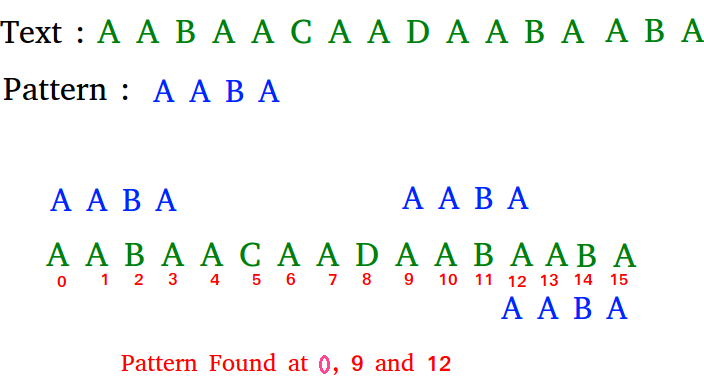# Naive algorithm for Pattern Searching

Given a text txt[0..n-1] and a pattern pat[0..m-1], write a function search(char pat[], char txt[]) that prints all occurrences of pat[] in txt[]. You may assume that n > m.

Examples:

```Input:  txt[] = "THIS IS A TEST TEXT"
pat[] = "TEST"
Output: Pattern found at index 10

Input:  txt[] =  "AABAACAADAABAABA"
pat[] =  "AABA"
Output: Pattern found at index 0
Pattern found at index 9
Pattern found at index 12```

Pattern searching is an important problem in computer science. When we do search for a string in notepad/word file or browser or database, pattern searching algorithms are used to show the search results.

Naive Pattern Searching:
Slide the pattern over text one by one and check for a match. If a match is found, then slides by 1 again to check for subsequent matches.

Implementation of above approach using CPP

// C++ program for Naive Pattern
// Searching algorithm
#include <bits/stdc++.h>
using namespace std;

void search(char* pat, char* txt)
{
int M = strlen(pat);
int N = strlen(txt);

/* A loop to slide pat[] one by one */
for (int i = 0; i <= N - M; i++) {
int j;

/* For current index i, check for pattern match */
for (j = 0; j < M; j++)
if (txt[i + j] != pat[j])
break;

if (j == M) // if pat[0...M-1] = txt[i, i+1, ...i+M-1]
cout << "Pattern found at index "
<< i << endl;
}
}

// Driver Code
int main()
{
char txt[] = "AABAACAADAABAAABAA";
char pat[] = "AABA";
search(pat, txt);
return 0;
}

Output:
```Pattern found at index 0
Pattern found at index 9
Pattern found at index 13 ```

What is the best case?
The best case occurs when the first character of the pattern is not present in text at all.

txt[] = "AABCCAADDEE";

pat[] = "FAA";

The number of comparisons in best case is O(n).

What is the worst case ?
The worst case of Naive Pattern Searching occurs in following scenarios.
1) When all characters of the text and pattern are same.

txt[] = "AAAAAAAAAAAAAAAAAA";

pat[] = "AAAAA";

2) Worst case also occurs when only the last character is different.

txt[] = "AAAAAAAAAAAAAAAAAB";

pat[] = "AAAAB";

The number of comparisons in the worst case is O(m*(n-m+1)). Although strings which have repeated characters are not likely to appear in English text, they may well occur in other applications (for example, in binary texts).#### More Articles of M Mounika:

Name Views Likes
C++ Segmented Sieve (Print Primes In a Range) 163 0
C++ Sieve Of Erastosthenes 136 0
C++ Gold Mine Problem 295 0
C++ Merge K Sorted Arrays 117 0
C++ K Centers Problem 240 0
C++ Find Nth Catalan Number 311 0
C++ Inplace Rotate square matrix by 90 degrees 286 0
C++ Find Non Repeating Elements in Array 87 0
C++ Merge Two Binary Trees 121 0
C++ Sum of Numbers From Root To Leaf Paths 89 0
C++ Meta Strings 92 0
C++ Flood Fill Algorithm 402 0
C++ smallest substring with maximum distinct characters 200 0
C++ Smallest window with all characters in string 94 0
C++ Minimum Removal of Characters from string to make its permutation as palindrome 87 0
C++ Minimum characters added at front of string in palindrome conversion 70 0
C++ Number of Bracket Reversals needed to make expression Balanced 73 0
C++ String to Palindrome with Append Function 83 0
C++ WildCard pattern matching 76 0
C++ Anagram substring Search 72 0
C++ Manachars Algorithm 74 0
C++ Search String in Grid 83 0
C++ String Matching(Z Algorithm) 67 0
C++ String Matching(Naive Algorithm) 115 0
C++ String Matching(KMP Algorithm) 141 0
C++ Remove Duplicates From String 111 0
C++ Basics of String Manipulation 85 1
C++ Disjoint Data Structure Cycle Detection 87 0
C++ Problem On Disjoint Data Structures 95 0
C++ Disjoint Data Structures Part3 79 0
Disjoint Data Structures Part2 91 0
Disjoint Data Structures 93 1
C++ Segment Trees 321 2
C++ Trie Cost of Data 291 1
C++ Trie Datastructure 279 1
C++ Greedy Approach Minimum number of coins 526 0
C++ Greedy Approach Maximum height Pyramid 329 1
C++ Greedy Approach String lexicographically largest subsequence 247 0
C++ Greedy Approach Lexicographically largest subsequence 364 0
C++ Greedy Approach Prims MST 398 1
C++ Greedy Approach Krushkals MST 458 1
C++ Greedy Approach N-array maximum sum 334 1
C++ Greedy Approach Policemen Catch Thieves 563 1
C++ Greedy Approach Maximum product Subset 546 1
C++ Greedy Approach Minimum Product Subset 349 1
C++ Greedy Approach Fractional Knapsack 737 1
C++ Greedy Approach-Activity Selection Problem 745 1
C++ Greedy Approach-Egyptian Fractions 640 0
C++ Greedy Approach-Huffman Codes 1031 1
C++ Introduction to Greedy Approach 955 2